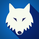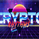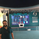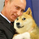# Strategy Code Example - Risk Management

54537 views
*** THIS IS JUST AN EXAMPLE OF STRATEGY RISK MANAGEMENT CODE IMPLEMENTATION ***

For my own future reference, and for anyone else who needs it.

Pine script strategy code can be confusing and awkward, so I finally sat down and had a little think about it and put something together that actually works (i think...)

Code is commented where I felt might be necessary (pretty much everything..) and covers:
• Take Profit
• Stop Loss
• Trailing Stop
• Trailing Stop Offset
...and details how to handle the input values for these in a way that allows them to be disabled if set to 0, without breaking the strategy.exit functionality or requiring a silly amount of statement nesting.

Also shows how to use functions (or variables/series) to execute trade entries and exits.

Cheers!
```//@version=2

strategy(title = "Strategy Code Example", shorttitle = "Strategy Code Example", overlay = true, pyramiding = 0, default_qty_type = strategy.percent_of_equity, default_qty_value = 10, currency = currency.GBP)

// Revision:        1
// Author:          @JayRogers
//
// *** THIS IS JUST AN EXAMPLE OF STRATEGY RISK MANAGEMENT CODE IMPLEMENTATION ***

// === GENERAL INPUTS ===
// short ma
maFastSource   = input(defval = open, title = "Fast MA Source")
maFastLength   = input(defval = 14, title = "Fast MA Period", minval = 1)
// long ma
maSlowSource   = input(defval = open, title = "Slow MA Source")
maSlowLength   = input(defval = 21, title = "Slow MA Period", minval = 1)

// === STRATEGY RELATED INPUTS ===
// the risk management inputs
inpTakeProfit   = input(defval = 1000, title = "Take Profit", minval = 0)
inpStopLoss     = input(defval = 200, title = "Stop Loss", minval = 0)
inpTrailStop    = input(defval = 200, title = "Trailing Stop Loss", minval = 0)
inpTrailOffset  = input(defval = 0, title = "Trailing Stop Loss Offset", minval = 0)

// === RISK MANAGEMENT VALUE PREP ===
// if an input is less than 1, assuming not wanted so we assign 'na' value to disable it.
useTakeProfit   = inpTakeProfit  >= 1 ? inpTakeProfit  : na
useStopLoss     = inpStopLoss    >= 1 ? inpStopLoss    : na
useTrailStop    = inpTrailStop   >= 1 ? inpTrailStop   : na
useTrailOffset  = inpTrailOffset >= 1 ? inpTrailOffset : na

// === SERIES SETUP ===
/// a couple of ma's..
maFast = ema(maFastSource, maFastLength)
maSlow = ema(maSlowSource, maSlowLength)

// === PLOTTING ===
fast = plot(maFast, title = "Fast MA", color = green, linewidth = 2, style = line, transp = 50)
slow = plot(maSlow, title = "Slow MA", color = red, linewidth = 2, style = line, transp = 50)

// === LOGIC ===
// is fast ma above slow ma?
aboveBelow = maFast >= maSlow ? true : false
// are we inverting our trade direction?
tradeDirection = tradeInvert ? aboveBelow ? false : true : aboveBelow ? true : false

// === STRATEGY - LONG POSITION EXECUTION ===
enterLong() => not tradeDirection and tradeDirection // functions can be used to wrap up and work out complex conditions
strategy.entry(id = "Long", long = true, when = enterLong()) // use function or simple condition to decide when to get in
strategy.close(id = "Long", when = exitLong()) // ...and when to get out
// === STRATEGY - SHORT POSITION EXECUTION ===
strategy.entry(id = "Short", long = false, when = enterShort())
strategy.close(id = "Short", when = exitShort())

// === STRATEGY RISK MANAGEMENT EXECUTION ===
// finally, make use of all the earlier values we got prepped
strategy.exit("Exit Long", from_entry = "Long", profit = useTakeProfit, loss = useStopLoss, trail_points = useTrailStop, trail_offset = useTrailOffset)
strategy.exit("Exit Short", from_entry = "Short", profit = useTakeProfit, loss = useStopLoss, trail_points = useTrailStop, trail_offset = useTrailOffset)
```how will you create alerts on
strategy.exit("Exit Long", from_entry = "Long", profit = useTakeProfit, loss = useStopLoss, trail_points = useTrailStop, trail_offset = useTrailOffset)
strategy.exit("Exit Short", from_entry = "Short", profit = useTakeProfit, loss = useStopLoss, trail_points = useTrailStop, trail_offset = useTrailOffset)

as those are functions of strategy.exit, right?Thanks a Ton man, i will remember you in my whole life..this post is my turning point in my trading journey. God Bless you!dysrupt
@dysrupt, trailing stop creates incorrect backtest results, no matter what method you use to determine it. if you are exiting with trailing stop strategy condition its no good (skews results positive in a huge way)theheirophant
@theheirophant, thanks for the reply. My thought process is, if the condition is "close<stop", in theory, the candle close would be the exact point of order close. Unless the stop was based on a higher resolution, resulting in major re-painting issues.